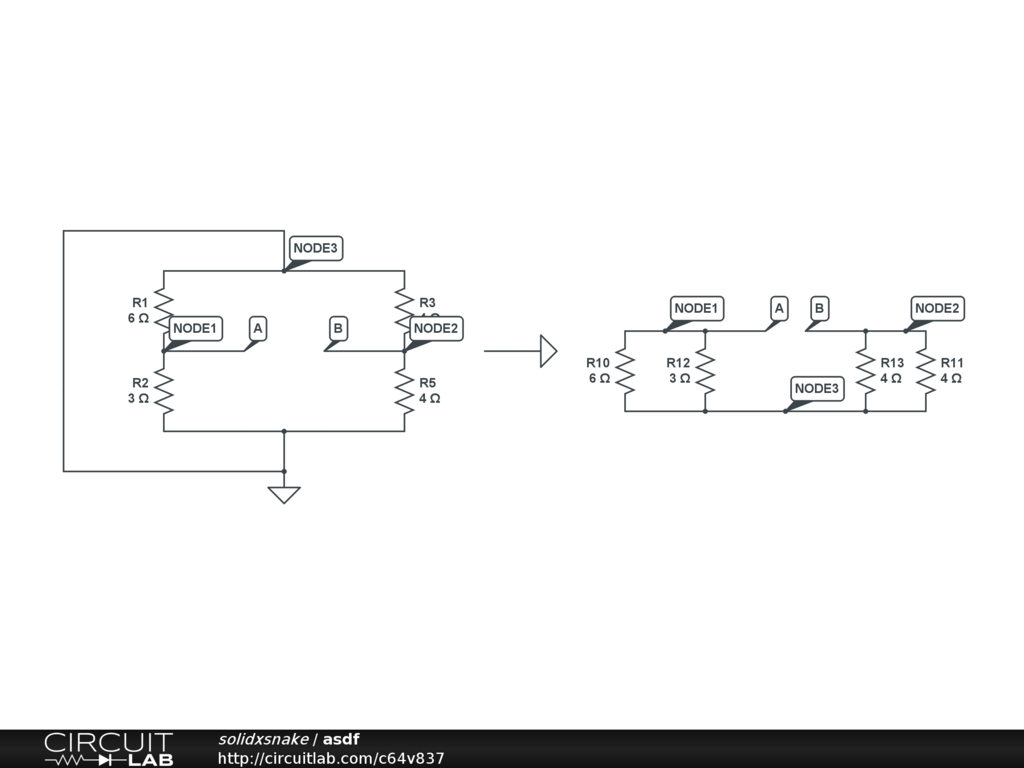# Total resistance of circuit

Hi I'm struggling a bit with obtaining the total resistance of this circuit, I know it's an easy one I'm just a bit confused on how the resistors are merged using parallel and series operations, here's an image:The overall goal is to obtain Thevenin's equivalent circuit so when obtaining $R_{th}$ the circuit is open between nodes A and B, and the voltage source is shorted out.

I've tried

• First
• Rp = R1//R3
• Rs = Rp + R2
• Rt = Rs//R4 = 2.29
• Second
• Rs1 = R1 + R2 = 9
• Rs2 = R3 + R4 = 8
• Rt = Rs1//Rs2 = 4.23

Any help is really appreciated.• thx for replying, According, to your diagram, $R_{th}$ should be 1 ohms? – Tristian Jun 4 '12 at 6:54